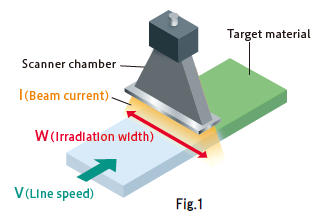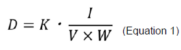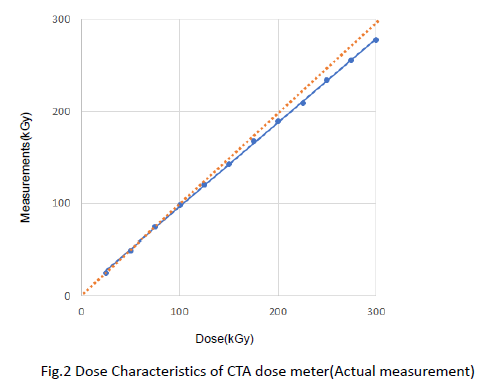## Useful information for Electron Beam

### Absorbed dose and Dosimetry

Posted date:April 27, 2023Last updated:April 27, 2023

Explanation of the concept of Absorbed dose and Dosimetry.

#### What is absorbed dose?

The important parameters for electron irradiation are acceleration voltage and absorbed dose, which are determined according to the application.
The amount of energy obtained by a material per unit mass due to irradiation is called dose, and the amount absorbed by a material is called absorbed dose.
The absorbed dose represents the degree of effect on the irradiated material; the higher the dose, the greater the effect on the material. The absorbed dose is the amount of energy given per unit mass, and is expressed in 1Gy =1 J/kg. Absorbed dose is also commonly used in units of kGy.
The absorbed dose per unit time is called the dose rate.

#### Relationship between Beam current and Line speed

A schematic diagram of electron irradiation is shown in Figure 1. The important parameters in considering the dose are the beam current, the line speed of the irradiated materials, and the irradiation width.
The relationship between these parameters can be expressed by the formula shown in (Equation 1).
K is an equipment-specific constant, and the dose is proportional to the beam current value and inversely proportional to the line speed and irradiation width. If the dose and processing speed required for irradiation are known, the required beam current value can be determined, which is useful in selecting the specifications of the equipment.D : Absorbed dose (kGy)
I  : Beam current (mA)
V : Line speed (m/min)
K : Constant

#### Dosimetry Methods

Dosimetry is used for the development and quality control of irradiated materials. This section describes dosimetry using CTA dose meter. The CTA dose meter is a colorless, transparent film that can be used to measure the dose range of electron-beam processing. The measurement principle is based on the fact that absorbance increases linearly with electron irradiation, and the dose is determined by measuring absorbance with a spectrophotometer or a dedicated measuring instrument.
Figure 2 shows the actual measurements of dosimetry using CTA dose meter, which deviates from the linear relationship above 100 kGy and should be used below that level.The absorbance of the CTA dose meter tends to increase slowly with the passage of time after irradiation, but the rate of increase becomes slower and slower. However, since the absorbance shows a fast fall during the 10 to 20 minutes immediately after irradiation, it is desirable to measure the absorbance 2 hours after irradiation.

#### Summary

Absorbed dose is one of the most important parameters in electron irradiation.
In this article, we explained that absorbed dose is the amount of energy given per unit mass, which is proportional to the beam current value and inversely proportional to the line speed and irradiation width. We also introduced CTA dose meter as an example of dosimetry. There are various other dosimeters available, but it is important to understand the characteristics of each one and to measure it under the correct environment.

Reference
「Manual of CTA Dose Meter」JAERI-M 82-033 (1982)
Ryūichi TANAKA  et al.

［Inquiry］

NHV Corporation
Please inquire through the inquiry form.